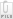Pay results fee

Sorry, you have not enough credits to pay results fee.

# Calculate percent of visible sky from flat surface

Launcher Erik Montes United States
Status Vaulted: May 24, 2011 04:16

### Labels

Geophysics Applied mathematics

### Description

Assuming one is standing on a flat surface with no obstructions (no trees, no buildings, etc.) on planet Earth, I would like to find out what is the percentage of sky visible. Additionally, I would like a formula that allows you to calculate the percentage of visible sky depending on altitude. See earth.png attachment below for additional details.earth.png

### Zomb Results

Results for this zomb have been placed in the vault.

You can access it for free.

sin theta = r_earth / (r_earth + altitude)

theta = arcsin( r_earth/(r_earth + altitude))

p_visible = (2pi - 2theta)/(2pi)*

p_visible = 1 - arcsin ( r_earth / (r_earth + altitude))/pi

Sanity checks:

r_earth = 6378.11000 m*

altitude = 1m

p_visible = .50006

altitude = 10^7 m

p_visible = .66766

This fits intuitively: the further away you are from the Earth's surface, the more of the sky you can see without the horizon interfering.

Python Script

import math

# Constants:
r_earth = 6378.1*1000

def p_visible(altitude):
'''
Returns the proportion of the sky visible.
altitude -- meters above Earth.
'''
return 1 - math.asin(r_earth/(r_earth + altitude))/math.picalculate-percent-of-visible-sky-from-flat-surface.png
Comments for results

No comments yet.

### Clarifications

Q:

Should the calculation include refraction caused by the earth's atmosphere? Please see http://en.wikipedia.org/wiki/Atmospheric_refraction and the diagram of the sun on the right-hand side.

Asked by benedict on May 23, 2011

A:

Yes, it should take refraction into consideration.

Q:

One more question - Can we assume that the earth is a sphere and that the atmosphere is also the same thickness at all latitudes (In fact the atmosphere is thicker at the equator due to rotation of the earth)? The atmosphere also varies in density depending on your altitude which makes it tricky: http://en.wikipedia.org/wiki/Atmosphere_of_Earth

• 50% of the atmosphere by mass is below an altitude of 5.6 km (18,000 ft).
• 90% of the atmosphere by mass is below an altitude of 16 km (52,000 ft). The common altitude of commercial airliners is about 10 km (33,000 ft) and Mt. Everest's summit is 8,848 m (29,029 ft) above sea level.
• 99.99997% of the atmosphere by mass is below 100 km (62 mi; 330,000 ft), although in the rarefied region above this there are auroras and other atmospheric effects. The highest X-15 plane flight in 1963 reached an altitude of 108.0 km (354,300 ft).

Asked by benedict on May 23, 2011

A:
No answer yet.
Disclaimer

Zombal does not in anyway guarantee the quality or correctness of the vaulted results. The results have been compiled by members on the site and have not been reviewed by a third party.

However, we encourage members to comment on vaulted work to improve its accuracy. If you're able to provide suggestions for improvement and to point out errors, you may be presented with the opportunity for paid follow-up work.